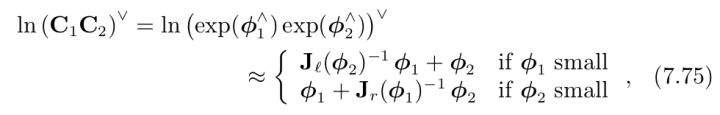## 四元数矩阵与 so(3) 左右雅可比

2018-05-22

${\bf q}({\boldsymbol \phi}) = [ \sin\frac{\phi}{2} {\bf a}, \, \cos \frac{\phi}{2}]'$, in which $\phi = \| \boldsymbol \phi\|, \, {\bf a} = \frac{\boldsymbol \phi}{\phi}$

### 四元数矩阵

$\frac{\partial {\bf e}_{\rm qt}}{\partial \boldsymbol \alpha} ={\bf Q}_l[{\bf q}({\bf e}_{\rm qt})] _{(1:3, 1:3)}$

### so(3) 雅可比### 参考文献

 S. Leutenegger, S. Lynen, M. Bosse, R. Siegwart, P. Furgale, “Keyframe-based visual-inertial odometry using nonlinear optimization”, Int. Journal of Robotics Research (IJRR), 2014.

 C. Forster, L. Carlone, F. Dellaert and D. Scaramuzza, “On-Manifold Preintegration for Real-Time Visual–Inertial Odometry,” in IEEE Transactions on Robotics, vol. 33, no. 1, pp. 1-21, Feb. 2017.

 T. Barfoot, State Estimation for Robotics.